# 8.2 Discussion and Exercises

The term scapegoat tree is due to Galperin and Rivest , who define and analyze these trees. However, the same structure was discovered earlier by Andersson [5,7], who called them general balanced trees since they can have any shape as long as their height is small.

Experimenting with the ScapegoatTree implementation will reveal that it is often considerably slower than the other SSet implementations in this book. This may be somewhat surprising, since height bound of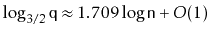is better than the expected length of a search path in a Skiplist and not too far from that of a Treap. The implementation could be optimized by storing the sizes of subtrees explicitly at each node or by reusing already computed subtree sizes (Exercises 8.5 and 8.6). Even with these optimizations, there will always be sequences of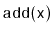and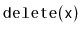operation for which a ScapegoatTree takes longer than other SSet implementations.

This gap in performance is due to the fact that, unlike the other SSet implementations discussed in this book, a ScapegoatTree can spend a lot of time restructuring itself. Exercise 8.3 asks you to prove that there are sequences of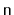operations in which a ScapegoatTree will spend on the order of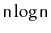time in calls to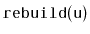. This is in contrast to other SSet implementations discussed in this book that only makestructural changes during a sequence ofoperations. This is, unfortunately, a necessary consequence of the fact that a ScapegoatTree does all its restructuring by calls to.

Despite their lack of performance, there are applications in which a ScapegoatTree could be the right choice. This would occur any time there is additional data associated with nodes that cannot be updated in constant time when a rotation is performed, but that can be updated during aoperation. In such cases, the ScapegoatTree and related structures based on partial rebuilding may work. An example of such an application is outlined in Exercise 8.12.

Exercise 8..1   Illustrate the addition of the values 1.5 and then 1.6 on the ScapegoatTree in Figure 8.1.

Exercise 8..2   Illustrate what happens when we start with an empty ScapegoatTree and add the sequence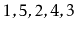and show where the credits described in the proof of Lemma 8.3 go, and how they are used during this sequence of additions.

Exercise 8..3   Show that, if we start with an empty ScapegoatTree and callfor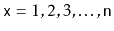, then the total time spent during calls tois at least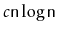for some constant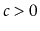.

Exercise 8..4   The ScapegoatTree, as described in this chapter, guarantees that the length of the search path does not exceed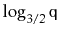.
1. Design, analyze, and implement a modified version of ScapegoatTree where the length of the search path does not exceed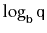, whereis a parameter with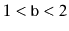.
2. What does your analysis and/or experiments say about the amortized cost of,andas a function ofand?

Exercise 8..5   Modify themethod of the ScapegoatTree so that it does not waste any time recomputing the sizes of subtrees that it has already computed the size of. This is possible because, by the time the method wants to compute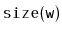, it has already computed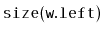or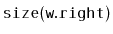. Compare the performance of your modified implementation with the implementation given here.

Exercise 8..6   Implement a second version of the ScapegoatTree data structure that explicitly stores and maintains the sizes of the subtree rooted at each node. Compare the performance of the resulting implementation with that of the original ScapegoatTree implementation as well as the implementation from Exercise 8.5.

Exercise 8..7   Reimplement themethod discussed at the beginning of this chapter so that it does not require the use of an array to store the nodes of the subtree being rebuilt. Instead, it should use recursion to first connect the nodes into a linked list and then convert this linked list into a perfectly balanced binary tree. (There are very elegant recursive implementations of both steps.)

Exercise 8..8   Analyze and implement a WeightBalancedTree. This is a tree in which each node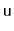, except the root, maintains the balance invariant that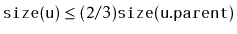. Theandoperations are identical to the standard BinarySearchTree operations, except that any time the balance invariant is violated at a node, the subtree rooted at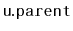is rebuilt. Your analysis should show that operations on a WeightBalancedTree run inamortized time.

Exercise 8..9   Analyze and implement a WeightBalancedTree. This is a tree in which each node, except the root, maintains the balance invariant that. Theandoperations are identical to the standard BinarySearchTree operations, except that any time the balance invariant is violated at a node, rotations are performed in order to restore these invariants. Your analysis should show that operations on a WeightBalancedTree run inworst-case time.

Exercise 8..10   Analyze and implement a CountdownTree. In a CountdownTree each nodekeeps a timer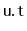. Theandoperations are exactly the same as in a standard BinarySearchTree except that, whenever one of these operations affects's subtree,is decremented. When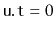the entire subtree rooted atis rebuilt into a perfectly balanced binary search tree. When a nodeis involved in a rebuilding operation (either becauseis rebuilt or one of's ancestors is rebuilt)is reset to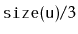.

Your analysis should show that operations on a CountdownTree run inamortized time. (Hint: First show that each nodesatisfies some version of a balance invariant.)

Exercise 8..11   Analyze and implement a DynamiteTree. In a DynamiteTree each nodekeeps tracks of the size of the subtree rooted atin a variable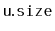. Theandoperations are exactly the same as in a standard BinarySearchTree except that, whenever one of these operations affects a node's subtree,explodes with probability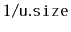. Whenexplodes, its entire subtree is rebuilt into a perfectly balanced binary search tree.

Your analysis should show that operations on a DynamiteTree run inexpected time.

Exercise 8..12   Design and implement a Sequence data structure that maintains a sequence (list) of elements. It supports these operations:
•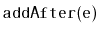: Add a new element after the element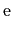in the sequence. Return the newly added element. (Ifis null, the new element is added at the beginning of the sequence.)
•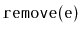: Removefrom the sequence.
•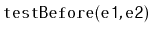: return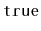if and only if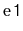comes beforein the sequence.
The first two operations should run inamortized time. The third operation should run in constant-time.

The Sequence data structure can be implemented by storing the elements in something like a ScapegoatTree, in the same order that they occur in the sequence. To implementin constant time, each elementis labelled with an integer that encodes the path from the root to. In this way,can be implemented just by comparing the labels ofand.

opendatastructures.org